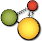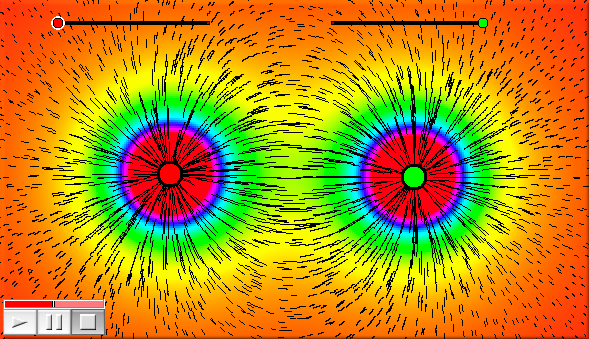# CindyJS## Interaction with CindyLab

#### The simulation environment: `simulation()`

Not available in CindyJS yet!

Description: This operator provides a handle to the simulation environment. The simulation environment offers several fields than can be used to access its global properties.

Name Writeable Type Purpose
`friction` yes real total friction of the simulation
`gravity` yes real total gravity of the simulation
`kinetic` no real total kinetic energy of the simulation
`ke` no real total kinetic energy of the simulation
`potential` no real total potential energy of the simulation
`pe` no real total potential energy of the simulation

#### Applying a force: `addforce(‹mass›,‹vec›)`

Not available in CindyJS yet!

Description: Applying a force `‹vec›` to an existing mass `‹mass›`. This operator is useful to implement user defined force fields. It should be called in the `Integration Tick` slot.

#### Applying a force: `setforce(‹mass›,‹vec›)`

Not available in CindyJS yet!

Description: Setting the force `‹vec›` for an existing mass `‹mass›`. This operator is very useful to implement user defined force fields. It should be called in the `Integration Tick` slot.

#### Probing particle forces: `force(‹vector›)`

Not available in CindyJS yet!

Description: The operator `force` is closely related to physics simulations in CindyLab. It can be used for testing the force that would affect a mass particle at a specific position. The vector `‹vector›` represents the position. The operator returns a two-dimensional vector that is the force at this position. If no modifiers are used, the operator assumes that the probe particle has `mass=1`, `charge=1` and `radius=1`.

Example: The following picture was generated using the `drawforces` operator and a color plot of the `force` operator. It shows the force field and force strength of the electrostatic field of two charges.```A.charge=(|C,G|-3)*3;
B.charge=(|E,H|-3)*3;
f(x):=max((0,min([x,1])));
colorplot([0.1,0.1,0.1]+hue(f(abs(force(#)/3))),(-10,-10),(20,10));
drawforces(stream->true,move->0.2,color->[0,0,0],resolution->10);```

Modifiers: It is also possible to set the values of mass, charge and radius explicitly. Each of these values can be set by a modifier of the same name. If at least one of these values is set explicitly, then all unset values are set to zero. Thus `force([0,0],charge->2)` tests the force that would be present for a particle of `charge=2`, `mass=0`, and `radius=0` at point `[0,0]`.